Probabilistic Programming

The programming languages and machine learning communities have, over the last few years, developed a shared set of research interests under the umbrella of probabilistic programming. The idea is that we might be able to “export” powerful PL concepts like abstraction and reuse to statistical modeling, which is currently an arcane and arduous task.

(You may want to read the most recent version of these lecture notes. The source is on GitHub. If you notice any mistakes, please open a pull request.)

## 1. What and Why

### 1.1. Probabilistic Programming is Not

Counterintuitively, probabilistic programming is not about writing software that behaves probabilistically. For example, if your program calls rand(3) as part of the work it's intended to doas in a cryptographic key generator, or an ASLR implementation in an OS kernel, or even a simulated-annealing optimizer for circuit designs)that's all well and good, but it's not what this topic is about.

It's best not to think of “writing software” at all. By way of analogy, traditional languages like C++, Haskell, and Python are obviously very different in philosophy, but you can imagine (if forced) using any of them to write, say, a cataloging system for your cat pictures or a great new alternative to LaTeX. One might be better for a given domain than the other, but they're all workable. Not so with probabilistic programming languages (PPL). It's more like Prolog: sure, it's a programming languagebut it's not the right tool for writing full-fledged software.

### 1.2. Probabilistic Programming Is

Instead, probabilistic programming is a tool for statistical modeling. The idea is to borrow lessons from the world of programming languages and apply them to the problems of designing and using statistical models. Experts construct statistical models alreadyby hand, in mathematical notation on paperbut it's an expert-only process that's hard to support with mechanical reasoning. The key insight in PP is that statistical modeling can, when you do it enough, start to feel a lot like programming. If we make the leap and actually use a real language for our modeling, many new tools become feasible. We can start to automate the tasks that used to justify writing a paper for each instance.

Here's a second definition: a probabilistic programming language is an ordinary programming language with rand and a great big pile of related tools that help you understand the program's statistical behavior.

Both of these definitions are accurate. They just emphasize different angles on the same core idea. Which one makes sense to you will depend on what you want to use PP for. But don't get distracted by the fact that PPL programs look a lot like ordinary software implementations, where the goal is to run the program and get some kind of output. The goal in PP is analysis, not execution.

### 1.3. An Example: Paper Recommendations

As a running example, let's imagine that we're building a system to recommend research papers to students based on the classes they take. To keep things simple, let's say there are only two research topics in the world: programming languages and statistics/machine learning. Every paper is either a PL paper, a statistics paper, or both. And we'll consider three courses at Cornell: CS 4110 (programming languages), CS 4780 (machine learning), and a fictional elective, “CS 4242,” on probabilistic programming.

It's pretty easy to imagine that machine learning should work for this problem: the mixture of areas revealed by your class schedule should say something about the papers you want to read. The problem is that the exact relationship can be hard to reason about directly. Clearly taking 4780 means you're more likely to be interested in statistics, but exactly how much more likely? What if you registered for 4780 because it was the only class that fit into your schedule? What do we do about people who only take the fictional CS 4242 and neither real coursedo we just assume they're 50/50 PL/stats people?

#### 1.3.1. Modeling the Problem

The machine-learning way of approaching this problem is to model the situation using random variables, some of which are latent. The key insight is that the arrows in Figure 1 don't make much sense: they don't really represent causality! It's not that taking 4110 makes you more interested in a given paper; there's some other factor that probabilistically causes both events. These are the latent random variables in a model for explaining the situation. Allowing yourself latent variables makes it much easier to reason directly about the problem.

Here's a model that introduces a couple of latent variables for each person's interest in statistics and programming languages. We'll get more specific about the model, but now the arrows at least make sense: they mean that one variable influences another in some way. Since we all know that you don't take every class you're interested in, we include a third hidden factor: how busy you are, which makes you less likely to go take any class.

This diagram of depicts a Bayesian network, which is a graph where each vertex is a random variable and each edge is a statistical dependence. Variables that don't have edges between them are statistically independent. (That is, knowing something about one of the variables tells you nothing about the outcome of the other.)

To complete the model, we'll also draw nodes and edges to depict how our latent interest variables affect paper relevance:

The idea isn't that we'll ask people what their interest levels and business are: we'll try to infer it from what we can observe. And then we can use this inferred information to do what we actually want to do: guess the paper relevance for a given student.

#### 1.3.2. A Model for Humans

So far, we've draw pictures of the dependencies in our model, but we need to get specific about what we mean. Here's how it normally goes: you write down a bunch of math that relates the random variables.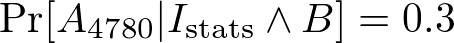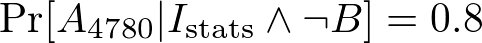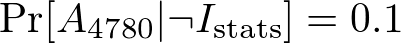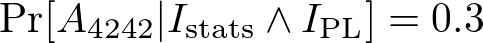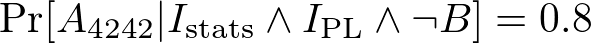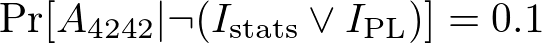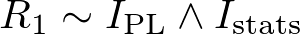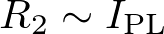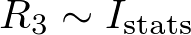That's a lot of math for such a simplistic model! And it's not even the hard part. The hardand usefulbit is statistical inference, where we guess the latent variables based on our observations. Statistical inference is a cornerstone of machine-learning research, and it's not easy. Traditionally, experts design bespoke inference algorithms for each new model they devise by hand.

Even this tiny example should demonstrate the drudgery of by-hand statistical modeling. It's like writing assembly code: we're doing something that feels a bit like programming, but there are no abstractions, no reuse, no descriptive variable names, no comments, no debugger, no type systems. Look at the equations for the class registration, for example: I got tired of writing out all that math because its so repetitive. This is clearly a job for an old-fashioned programming language abstraction: a function. The goal of PPLs is to bring the old and powerful magic of programming languages, which you already know and love, to the world of statistics.

## 2. Basic Concepts

To introduce the basic concepts of a probabilistic programming language, I'll use a project called webppl, which is a PPL embedded in JavaScript. You can read more about this language in The Design and Implementation of Probabilistic Programming Languages, an in-progress book by Noah Goodman and Andreas Stuhlmüller from Stanford. It's a nice language to use as an introduction because you can play with it right in your browser.

### 2.1. Random Primitives

The first thing that makes a language a probabilistic programming language (PPL) is a set of primitives for drawing random numbers. At this point, a PPL looks like any old imperative language with a rand call. Here's an incredibly boring webppl program:

var b = flip(0.5);
b ? "yes" : "no"


This boring program just uses the outcome of a fair coin toss to return one string or another. It works exactly like an ordinary program with access to a flip function for producing random Booleans. Functions like flip are sometimes called elementary random primitives, and they're the source of all randomness in these programs.

If you run this little program in the webppl editor, you'll see that it does exactly what you expect: sometimes it outputs “yes,” and sometimes it prints “no.”

Things get slightly more interesting when we realize that webppl can represent entire distributions, not just individual values. The webppl language has an Enumerate operation, which prints out all the probabilities in a distribution defined by a function:

var roll = function () {
var die1 = randomInteger(6) + 1;
var die2 = randomInteger(6) + 1;
return die1 + die2;
}

var dist = Enumerate(roll);
print(dist);
viz.auto(dist);


You get a printout with all the possible die values between 2 and 14 and their associated probabilities. That viz.auto call also instructs webppl's in-browser interface to display a pretty graph.

This may not look all that surprising, since you could imagine writing Enumerate in your favorite language by just running the roll function over and over. But in fact, Enumerate is doing something a bit more powerful. It's not sampling executions to get an approximation of the distribution; it's actually enumerating every possible execution of the function to get an exact distribution. This begins to reveal the point of a probabilistic programming language: the tools that analyze PPL programs are the important part, not actually executing the programs directly.

### 2.2. Our Example Model in webppl

This is enough to code up the math for our paper-recommender model. We can write functions to encode the relevance piece and the class registration piece, and we can test it out by randomly generating “student profiles.”

// Class attendance model.
var attendance = function(i_pl, i_stats, busy) {
var attendance = function (interest, busy) {
if (interest) {
return busy ? flip(0.3) : flip(0.8);
} else {
return flip(0.1);
}
}
var a_4110 = attendance(i_pl, busy);
var a_4780 = attendance(i_stats, busy);
var a_4242 = attendance(i_pl && i_stats, busy);

return {cs4110: a_4110, cs4780: a_4780, cs4242: a_4242};
}

// Relevance of our three papers.
var relevance = function(i_pl, i_stats) {
var rel1 = i_pl && i_stats;
var rel2 = i_pl;
var rel3 = i_stats;

return {paper1: rel1, paper2: rel2, paper3: rel3};
}

// A combined model.
var model = function() {
// Some even random priors for our "student profile."
var i_pl = flip(0.5);
var i_stats = flip(0.5);
var busy = flip(0.5);

return [relevance(i_pl, i_stats), attendance(i_pl, i_stats, busy)];
}

var dist = Enumerate(model);
viz.auto(dist);


Running this will show the distribution over all the observed data. This isn't terribly useful, but it is interesting. We can see, for example, that if we know nothing else about a student, our model says they're quite likely to take none of the classes and to be interested in none of the papers.

### 2.3. Conditioning

The next important piece of a PPL is a conditioning construct. Conditioning lets you determine how much to weight to give to a given execution in a program. Crucially, you can choose the weight of an execution partway through the runafter doing some of the computation. You can even mark an execution as completely irrelevant, effectively filtering the executions to a subset.

This conditioning operation is particularly useful for encoding observations. If you know something about the outcome of a process, you can use conditioning to communicate that the observation must be true (or is likely to be true). Let's return to our dice example for a contrived example. Say that we saw the first die, and it's a 4. We can condition on this information to give us the distribution on total values given that one of the dice is a 4:

var roll = function () {
var die1 = randomInteger(6) + 1;
var die2 = randomInteger(6) + 1;

// Only keep executions where at least one die is a 4.
if (!(die1 === 4 || die2 === 4)) {
factor(-Infinity);
}

return die1 + die2;
}

Unsurprisingly, the distribution is flat except for the outcome 8, which is less likely because only one 4-containing roll can produce that total. Outcomes like 2 and 12 have probability zero because they cannot occur when one of the dice comes up 4.

What if, on the other hand, we don't get to see either of the dice, but someone told us that the total on the dice was 10. What does this tell us about the values of the dice themselves? We can encode this observation by conditioning on the outcome of the roll.

var roll_condition = function () {
var die1 = randomInteger(6) + 1;
var die2 = randomInteger(6) + 1;

// Discard any executions that don't sum to 10.
var out = die1 + die2;
if (out !== 10) {
factor(-Infinity);
}

// Return the values on the dice.
return die1 + "+" + die2;
}

The results probably don't surprise you, but we can use the same principle with our recommender.

### 2.4. Actually Recommending Papers

Let's use the same philosophy now to actually produce recommendations. It's simple: we just need to condition on the class registration of the person we're interested in. Here's an example that describes me: I attend my own class, CS 4110, and the fictional PPL class, CS 4242, but not the ML class, 4780.

// A model query that describes my class attendance.
var rec = function() {
var i_pl = flip(0.5);
var i_stats = flip(0.5);
var busy = flip(0.5);

// Require my conference attendance.
var att = attendance(i_pl, i_stats, busy);
require(att.cs4242 && att.cs4110 && !att.cs4780);

return relevance(i_pl, i_stats);
}

(In this example, we define require to wrap factor(-Infinity) and completely eliminate program executions that don't satisfy a condition.) Calling Enumerate on this rec function finally gives us something useful: a distribution over paper relevance! It's a little easier to understand if we just look at one paper at a time:

return relevance(i_pl, i_stats).paper1;

Suddenly, this is pretty nifty! By telling the enumerator which executions are relevant to us, it can tell us what it knows about data under those conditions. To me, and I think to most programmers, this already feels like a much more natural tool for expressing probabilistic models. Rather than carefully writing down which random variables depend on which others, you use the flow of program execution to build up those dependencies.

The point of this section: Writing generative models feels very comfortable and straightforward, and a programming language is a great way to write down a generative algorithm. We've successfully made our job easy by shifting the burden of doing inference on these simple models to the compiler and tools.

### 2.5. Inference

That Enumerate operation might not look like much, but it's actually an implementation of the central problem that PPLs were meant to solve: statistical inference. Especially in the presence of conditioning, inference on a general probabilistic program is a hard problem. Think about how Enumerate must work: it needs to know the outcome of a program for every single valuation of the program's random primitive draws. It's not hard to see how this grows exponentially. And it's even less clear if you introduce floating-point numbers: if you draw a number between 0.0 and 1.0, that's a lot of possible valuationsand it's not representable as a histogram.

Enumerate just won't do for nontrivial problems. It's for this reason that efficient inference algorithms for PPLs are a huge focus in the community. Here are a couple of other inference algorithms that don't involve exploring every possible execution of a program.

#### 2.5.1. Rejection Sampling

The second most obvious inference algorithm uses sampling. The idea is to run the program a large number of times, drawing different random values for each random primitive on each execution. Apply the program's conditioning to weight each sample and total them all up. The interaction with weighting makes this strategy rejection sampling, so called because you reject some executions when you reach a conditioning statement.

The webppl language has this strategy built in. It's called ParticleFilter, and we can run it on our examples from above:

var sampled = ParticleFilter(rec('paper1'), 1000);

#### 2.5.2. MCMC

Rejection sampling works for small examples, but it runs intro trouble in the presence of conditioning. It can waste a lot of work taking samples that don't matter (i.e., they're destined to be rejected when they hit a factor call). Smarter sampling strategies exist, the most prominent of which are Markov chain Monte Carlo methods.

The webppl standard library provides MCMC algorithms. Making MCMC correct and efficient is a popular problem in PPL research, and a full discussion is currently out of scope of this lecture.

## 3. Applications

To be as starry-eyed as possible, the promise of PP is nothing less than the democratization of machine learning. In case you're not convinced yet, here are a sampling of popular applications of probabilistic programming.

• TrueSkill is perhaps the most widely cited example, originally laid out in this ESOP'11 paper. It's the model used by multiplayer Xbox games to find good matchups among players. The idea is to model latent variables for the player's skills and to match people up so that the predicted win probability is close to 50/50.
• The webppl book has a nifty computer vision demo that shows how a generative “forward” model can also be used “backward” to solve a complementary problem.
• Forest is a dizzying resource of generative models written in PPLs for domains from cognition and NLP to document retrieval.
• This is not quite an application yet, but Gamalon is a new startup founded by Ben Vigoda of Lyric Semiconductor, Noah Goodman, and others. It seems to be centered around probabilistic programming.
• Then there's my favorite application: understanding normal programs that deal with probabilities. There is a lot of ordinary software that deals with probabilistic behavior:
• approximate computing
• dealing with sensors
• security/obfuscation

## 4. Techniques

This section gives a few examples of recent work from the programming languages community that focuses on probabilistic programming.

### 4.1. Probabilistic Assertions

At the very beginning of the lecture, I said that probabilistic programming was not about writing real software that you actually want to execute. But some research has worked to apply the lessons of PPL research to ordinary programming with probabilistic behavior.

One project I worked on myself used this philosophy to help express correctness constraints for software that behaves statistically. The idea is to introduce a probabilistic assertion, written passert, to generalize the familiar assert statement to work probabilistically. The goals in that project are to:

• Work on messy programs in a real-world programming language (here, LLVM programs, so think C and C++).
• Make it fast to check statistical properties on the output. Think quality thresholds for approximate programs.
• Not care about conditioning. Regular software doesn't need factor statements, which simplifies the job of checking passerts.

### 4.2. R2

R2 is a probabilistic programming language and implementation from Microsoft. The tool is available for download.

One particularly nifty component of R2 is the application of classic PL ideas to improve statistical inference. Most prominently, the R2 people use weakest preconditions analysis to improve rejection samplingthat naive randomized inference strategy we talked about before.

The WP approach addresses the most frustrating aspect of conditioning: the fact that you find out after doing a bunch of work that you have to throw it all away. In this passage from earlier:

var die1 = randomInteger(6) + 1;
var die2 = randomInteger(6) + 1;

// Discard any executions that don’t sum to 10.
var out = die1 + die2;
require(out === 10);

we condition late in the program, which is inefficient if we use a naive strategy. (Bear with me here and pretend that the addition in the second-to-last line is expensive.) We can improve this program by making the assertion earlier in the program. And, in fact, that is the essence of a WP analysis, which asks, What must be true at program point A in order to make a different property true at a later point B? In our example, we can “move up” the condition to fail faster:

var die1 = randomInteger(6) + 1;
var die2 = randomInteger(6) + 1;

require((die1 == 3 && die2 == 7) || ...);
var out = die1 + die2;

If we're lucky, we can even move the condition all the way back into the primitive sampling calland avoid doing any work at all that will eventually be rejected later.

### 4.3. Porting PL Ideas to PP

Part of the promise of probabilistic programming is that we should be able to “port” ideas from the standard PL literature to the PPL world. Here are some examples: